# Basis Vectors in Linear Algebra – ML

• Difficulty Level : Medium
• Last Updated : 13 Dec, 2021

For understanding the concept behind Machine Learning, as well as Deep Learning, Linear Algebra principles, are crucial. Linear algebra is a branch of mathematics that allows to define and perform operations on higher-dimensional coordinates and plane interactions in a concise way. Its main focus is on linear equation systems.

• Idea behind basis vector?
• Definition of basis vector
• Properties of basis vector
• Basis vectors for a given space
• It’s important from a data science viewpoint

What’s the idea behind basis vectors?
So, the idea here is the following,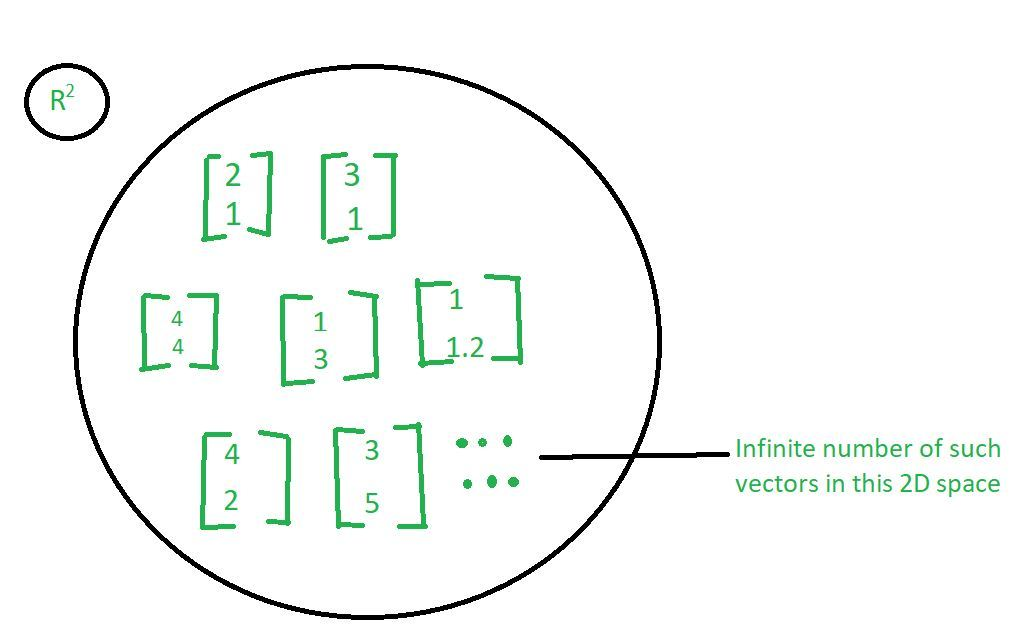Let us take an R-squared space which basically means that, we are looking at vectors in 2 dimensions. It means that there are 2 components in each of these vectors as we have taken in the above image. We can take many many vectors. So, there will be an infinite number of vectors, which will be in 2 dimensions. So, the point is can we represent all of these vectors using some basic elements and then some combination of these basic elements.

Now, let us consider 2 vectors for example,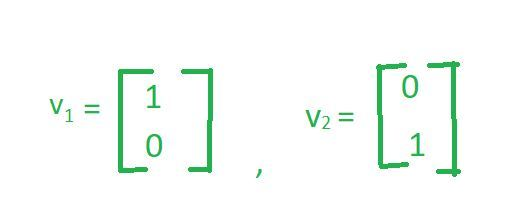Now, if you take any vector that given in R squared space, let us say take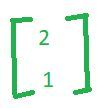We can write this vector as some linear combination, of this vector plus this vector as follows.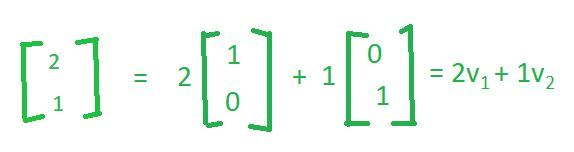Similarly, if you take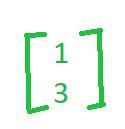We can also write this vector as some linear combination, of this vector plus this vector as follows.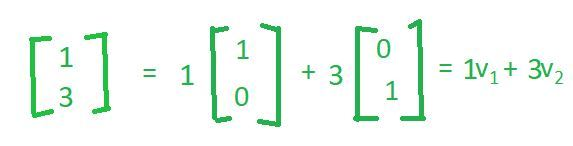Similarly,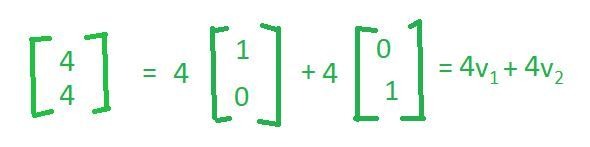And that would be true for any vector that you have in this space.

So, in some sense what we say is that these 2 vectors(v1 and v2) characterize the space or they form a basis for space and any vector in this space, can simply be written as a linear combination of these 2 vectors. Now you can notice, the linear combinations are actually the numbers themselves. So, for example, if I want vector(2, 1) to be written as a linear combination of the vector(1, 0) and vector(0, 1), the scalar multiples are 2 and 1 which is similarly for vector(4, 4) and so on.

So, the key point is while we have an infinite number of vectors here, they can all be generated as a linear combination of just 2 vectors and we have seen here that these 2 vectors are vector(1, 0) and vector(0, 1). Now, these 2 vectors are called the basis for the whole space.

Definition of basis vector: If you can write every vector in a given space as a linear combination of some vectors and these vectors are independent of each other then we call them as basis vectors for that given space.

Properties of basis vector:

1. Basis vectors must be linearly independent of each other:
If I multiply v1 by any scalar, I will never be able to get the vector v2. And that proves that v1 and v2 are linearly independent of each other. We want basis vectors to be linearly independent of each other because we want every vector, that is on the basis to generate unique information. If they become dependent on each other, then this vector is not going to bring in anything unique.

2. Basis vectors must span the whole space:
The word span basically means that any vector in that space, I can write as a linear combination of the basis vectors as we see in our previous example.

3. Basis vectors are not unique: One can find many many sets of basis vectors. The only conditions are that they have to be linearly independent and should span the whole space. So let’s understand this property in detail by taking the same example as we have taken before.

Let us consider 2 other vectors, which are linearly independent of each other.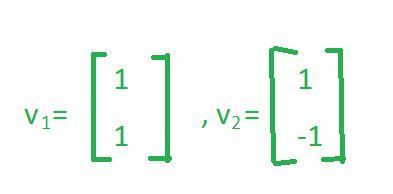• First we have to check are these 2 vectors obeying the properties of basis vector?
You can see that these 2 vectors are linearly independent of each other as multiplying v1 by any scalar never able to get the vector v2. So, for example, if I multiply v1 by -1 I will get vector(-1, -1), but not the vector(1, -1)

To verify the second property, let’s take the vector(2, 1). Now, let us see whether we can represent this vector(2, 1) as a linear combination of the vector(1, 1) and vector(1, -1)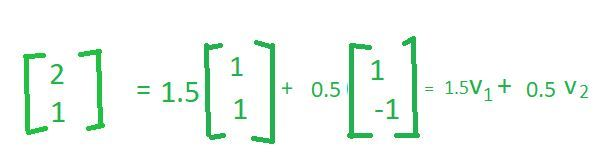• So, if you take a look at this we have successfully represented this vector(2, 1) as a linear combination of the vector(1, 1) and vector(1, -1). You can notice that in the previous case when we use the vector(1, 0) and vector(0, 1), we said this can be written as 2 times of vector(1, 0) and 1 time of vector(0, 1); however, the numbers have changed now. Nonetheless, I can write this as a linear combination of these 2 basis vectors.

Similarly, if you take the vector(1,3)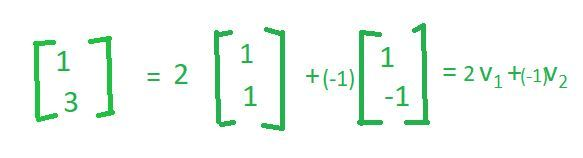• Similarly, if you take the vector(4,4)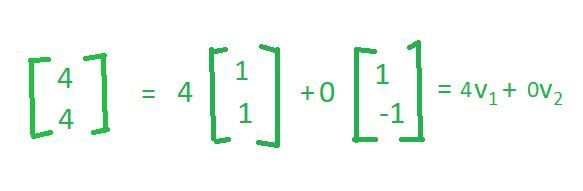• So, this is another linear combination of the same basis vectors. So, the key point that I want to make here is that the basis vectors are not unique. There are many ways in which you can define the basis vectors; however, they all share the same property that, if I have a set of vectors which I call as a basis vector, those vectors have to be independent of each other and they should span the whole space.

Hence, this v1 and v2 are also basis vectors for R2.

Point to remember:
An interesting thing to note here is that we cannot have 2 basis sets which have a different number of vectors. What I mean here is in the previous example though the basis vectors were v1(1, 0) and v2(0, 1) there were only 2 vectors. Similarly, in this case, the basis vectors are v1(1, 1) and v2(1, -1). However, there are still only 2 vectors. So, while you could have many sets of basis vectors, all of them being equivalent to the number of vectors in each set will be the same, they cannot be different. So something that you should keep in mind that for the same space you can not have 2 basis sets one with n vectors and another one with m vectors that is not possible. So, if it is a basic set for the same space, the number of vectors in each set should be the same.

Find basis vectors:

Let’s take an example of R4 space. What it actually means that there are 4 components in each of these vectors.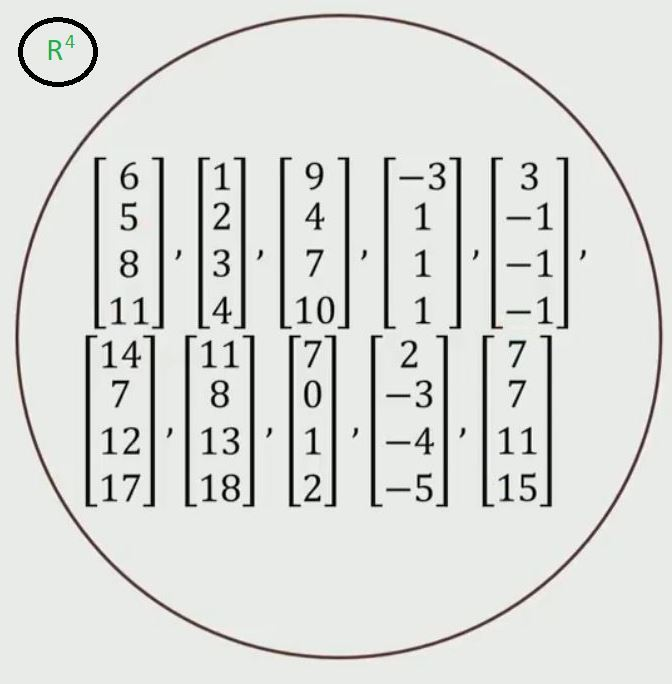• Step 1: To find basis vectors of the given set of vectors, arrange the vectors in matrix form as shown below.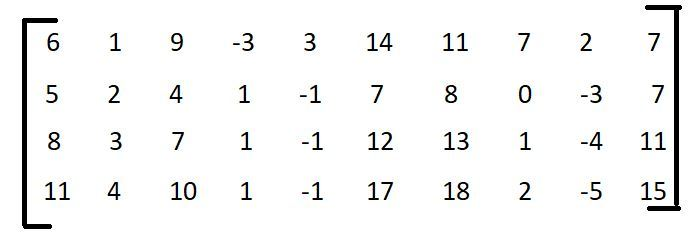• Step 2: Find the rank of this matrix.
If you identify the rank of this matrix it will give you the number of linearly independent columns. The rank of the matrix will tell us, how many are fundamental to explaining all of these columns, and how many columns do we need. So, that we can generate the remaining columns as a linear combination of these columns.

To find out the rank of matrix please refer this link. So for this, the rank of the matrix is 2.

• Step 3:
Any two independent columns can be picked from the above matrix as basis vectors.

Explanation:
If the rank of the matrix is 1 then we have only 1 basis vector, if the rank is 2 then there are 2 basis vectors if 3 then there are 3 basis vectors and so on. In this case, since the rank of the matrix turns out to be 2, there are only 2 column vectors that I need to represent every column in this matrix. So, the basis set has size 2. So, we can pick any 2 linearly independent columns here and then those could be the basis vectors.

So, for example, we could choose v1(6, 5, 8, 11) and v2(1, 2, 3, 4) and say, this is the basis vector for all of these columns or we could choose v1(3, -1, -1, -1) and v2(7, 7, 11, 15) and so on. We can choose any 2 columns as long as they are linearly independent of each other and this is something that we know from above that the basis vectors need not be unique. So, I pick any 2 linearly independent columns that represent this data.

Important from a data science viewpoint
Now, let me explain to you why this basis vectors concept is very very important from a data science viewpoint. Just take a look at the previous example. We have 10 samples and we want to store these 10 samples since each sample has 4 numbers, we would be storing 4 x 10 = 40 numbers.
Now, let us assume we do the same exercise, for these 10 samples and then we find that we have only 2 basis vectors, which are going to be 2 vectors out of this set. What we could do is, we could store these 2 basis vectors that, would be 2 x 4 = 8 numbers and for the remaining 8 samples, instead of storing all the samples and all the numbers in each of these samples, what we could do is for each sample we could just store 2 numbers, which are the linear combinations that we are going to use to construct this. So, instead of storing these 4 numbers, we could simply store those 2 constants and since we already have stored the basis vectors, whenever we want to reconstruct this, we can simply take the first constant and multiply it by v1 plus the second constant multiply it by v2 and we will get this number.

So in summary,

We store 2 basis vectors which give me: 4 x 2 = 8 numbers
And then for the remaining 8 samples, we simply store 2 constants e.g: 8 x 2 = 16 numbers
So, this would give us: 8 + 16 = 24 numbers
Hence instead of storing 4 x 10 = 40 numbers, we can store only 24 numbers, which is the approximately half reduction in number. And we will be able to reconstruct the whole data set by storing only 24 numbers.

So, for example, if you have a 30-dimensional vector and the basis vectors are just 3, then you can see the kind of reduction, that you will get in terms of data storage. So, this is one viewpoint of data science.

Why the reduction in data storage going to benefit from a data science viewpoint?
It is very important to understand and characterize the data in terms of what fundamentally characterizes the data. So, that you can store less, we can do smarter computations and there are many other reasons why we will want to do this,

• You can identify this basis to identify a model between this data.
• You can identify a basis to do noise reduction in the data.

My Personal Notes arrow_drop_up